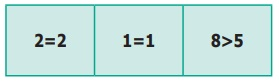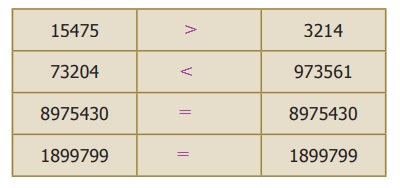Home | | Maths 6th Std | Comparison of Numbers

# Comparison of Numbers

We are familiar with the concept of comparing numbers and finding the biggest among them. We use symbols <, > and = to compare any two numbers.

Comparison of Numbers

We are familiar with the concept of comparing numbers and finding the biggest among them. We use symbols <, > and = to compare any two numbers.

1. Comparing numbers with unequal number of digits

1. When we compare two numbers 16090 and 100616, we have already learnt that the number with more digits is greater.

Hence, 1,00,616 (6 digit number) > 16,090 (5 digit number).

2. Suppose we are given more than two numbers say 1468, 5, 201, 69 and 70000. Then among these, we can immediately say that the number 70000 is the greatest and 5 is the least, based on the number of digits.

Try these

1. Write the numbers in the ascending order: 688, 9, 23005, 50, 7500.

Solution: Ascending order 9, 50, 688, 7500, 23005

2. Find the least and the greatest among the numbers: 478, 98, 6348, 3, 6007, 50935.

Solution:

least number = 3

greatest number = 50935

2. Comparing numbers with equal number of digits

In a distance analysis chart, the distance between Chennai and New Delhi is 2180 km and Chennai to Noida is 2158 km respectively. Which city is farther from Chennai?Compare the given numbers 2180 and 2158 using the above mentioned steps.Hence, 2180 > 2158.

Example 1.4

Compare 59283746 and 59283748 using place value chart.

Solution

Step 1: Number of digits in the two given numbers are equal.

Step 2: Compare the place values using the place value chart.Compare the digits of the two numbers from the highest place value as noted below.

5 = 5, 9 = 9, 2 = 2, 8 = 8, 3 = 3, 7 = 7, 4 = 4, 6 < 8

Here only the digits in the ones place are not equal and 6 < 8.

Hence, 59283746 < 59283748.

Try these

Compare the two numbers and put <, > and = using place value chart.The number

10100 is called googol (this is, 10 multiplied 100 times)

The number 10googol = 10(10100) is called googolplex

Tags : Numbers | Term 1 Chapter 1 | 6th Maths , 6th Maths : Term 1 Unit 1 : Numbers
Study Material, Lecturing Notes, Assignment, Reference, Wiki description explanation, brief detail
6th Maths : Term 1 Unit 1 : Numbers : Comparison of Numbers | Numbers | Term 1 Chapter 1 | 6th Maths